﻿ 深沪股市的波动率分析——基于GARCH和SV模型 Volatility Analysis of Shenzhen and Shanghai Stock Markets—Based on GARCH and SV Models

Vol. 08  No. 08 ( 2019 ), Article ID: 31852 , 10 pages
10.12677/ASS.2019.88200

Volatility Analysis of Shenzhen and Shanghai Stock Markets—Based on GARCH and SV Models

Hanqi Qin

Jiangxi University of Finance and Economics, Nanchang Jiangxi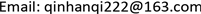Received: Aug. 2nd, 2019; accepted: Aug. 16th, 2019; published: Aug. 23rd, 2019ABSTRACT

This paper selects the closing prices of Shanghai Composite Index and Shenzhen Composite Index from January 2016 to May 2019 as the original data, calculates the return rates of the two indexes, and analyzes the fluctuation of the stock return rates in the two cities. Through the establishment of GARCH model and SV model, the results show that GARCH model and SV model can well describe and fit the fluctuation of the return rate in China's stock market, and through EGARCH test, it is found that the return rate has leverage effect. Therefore, some suggestions are put forward to stabilize the fluctuation intensity of the stock market and reduce the risk of the stock market.

Keywords:Volatility of the Rate of Return, GARCH Model, SV Model1. 前言

2019年5月11日，证监会主席易会满出席中国上市公司协会2019年年会暨第二届理事会第七次会议并发表讲话中提到：近期A股面临着大幅波动的情况，股市有一定的震荡波动。资本市场是实体经济的“晴雨表”，最近一段时间以来中国股市出现了明显的波动和下滑，这是由诸多因素造成的。在我国金融体系中，由于市场形势瞬息万变，牵动着股市市场随之变化和发展。我国股票市场的波动强烈是有目共睹的，因此分析我国股市问题迫在眉睫，将如何采取最合适的手段和方法来进行有效地刻画股票市场的波动以及衡量它们的风险？

2. GARCH模型

2.1. ARCH模型

${\epsilon }_{t}^{2}={\alpha }_{0}+{\alpha }_{1}{\epsilon }_{t-1}^{2}+\cdots +{\alpha }_{p}{\epsilon }_{t-q}^{2}+{\eta }_{t}$ (1)

2.2. GARCH模型

GARCH与ARCH模型较为相似，是建立在ARCH模型的基础上，一种广义的表达，GARCH模型通常用于对回归或自回归模型的随机扰动项进行建模。若ARCH模型可以写成下面的形式：

${h}_{t}={\alpha }_{0}+{\alpha }_{1}{\epsilon }_{t-1}^{2}+\cdots +{\alpha }_{q}{\epsilon }_{t-q}^{2}+{\theta }_{1}{h}_{t-1}+\cdots +{\theta }_{p}{h}_{t-p}$ (2)

${h}_{t}={\alpha }_{0}+\underset{i=1}{\overset{q}{\sum }}{\alpha }_{i}{\epsilon }_{t-i}^{2}+\underset{i=1}{\overset{q}{\sum }}{\theta }_{j}{h}_{t-j}^{2}$ (3)

3. SV-t模型

${R}_{t}={\epsilon }_{t}{\text{e}}^{\frac{{h}_{t}}{2}},\text{\hspace{0.17em}}\text{\hspace{0.17em}}{\epsilon }_{t}~\text{i}\text{.i}\text{.d}t\left(\text{0,1,}\omega \right)$ (4)

${h}_{t}=\mu +\phi \left({h}_{t-1}-\mu \right)+{\gamma }_{t},\text{\hspace{0.17em}}{\gamma }_{t}~N\left(0,{\sigma }^{2}\right)$ (5)

4. 实证分析

4.1. 数据的选择和处理

${R}_{h,t}=\mathrm{ln}{h}_{t}-\mathrm{ln}{h}_{t-1}=\mathrm{ln}\left(\frac{{h}_{t}}{{h}_{t-1}}\right)$ (6)

${R}_{z,t}=\mathrm{ln}{z}_{t}-\mathrm{ln}{z}_{t-1}=\mathrm{ln}\left(\frac{{z}_{t}}{{z}_{t\text{-}1}}\right)$ (7)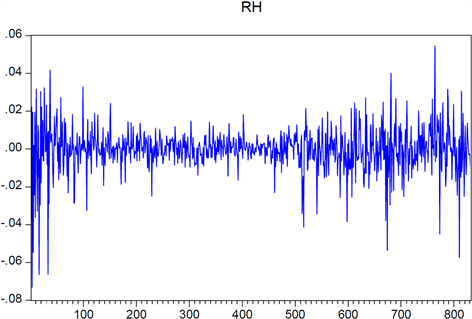Figure 1. Return sequence diagram of shanghai composite index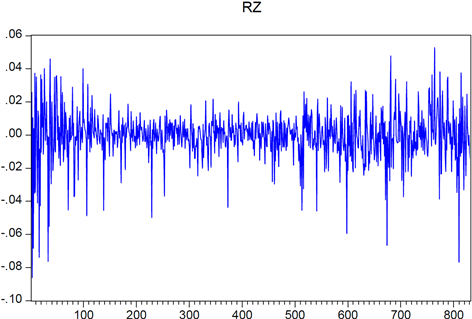Figure 2. Shenzhen composite index return sequence chart

4.2. 数据描述性分析

4.3. 考察序列的平稳性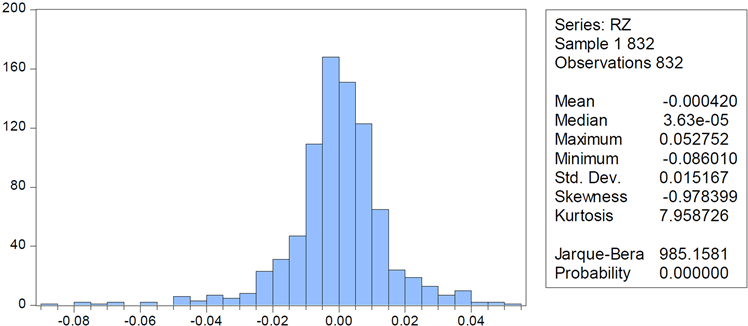Figure 3. Descriptive chart of shanghai composite index return rate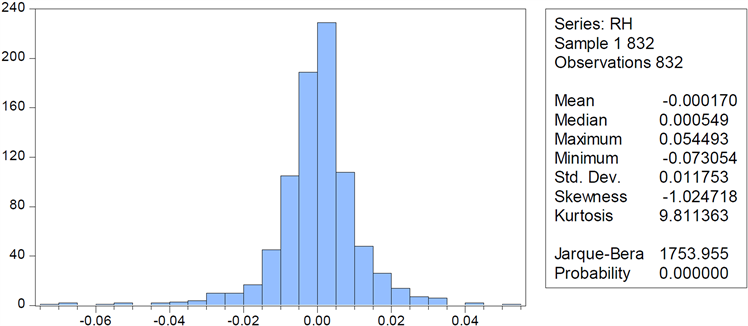Figure 4. Descriptive statistic of the Shenzhen Composite Index’s yield

4.4. 序列的ARCH-LM效应检验

4.5. 序列的自相关以及偏自相关检验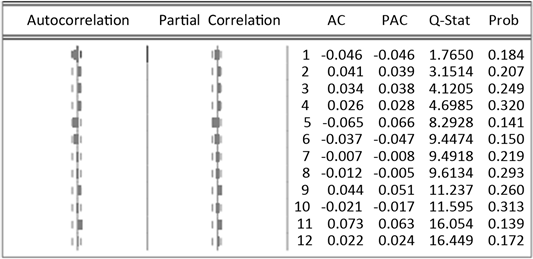Figure 5. Correlation test result chartFigure 6. QQ chart of return rate of shanghai composite index and Shenzhen composite index

4.6. GARCH模型实证Table 4. Comparison of test results of three modelsTable 5. Parameter estimation of Shenzhen composite index return seriesTable 6. ARCH-M test results of Shenzhen composite index return sequenceTable 7. Table of estimated results for different orders of Shenzhen composite index return seriesTable 8. Parameter estimation of Shenzhen composite index return series

4.7. SV模型实证

$\begin{array}{l}\mu \sim N\left(0,100\right)\\ {\phi }_{1}\sim Beta\left(20,1.5\right)\\ \tau \sim Gamma\left(2.5,0.025\right)\\ \omega \sim {\chi }^{2}\left(8\right)\end{array}$Table 9. Estimation results of parameters of MCMC algorithm in SV-T model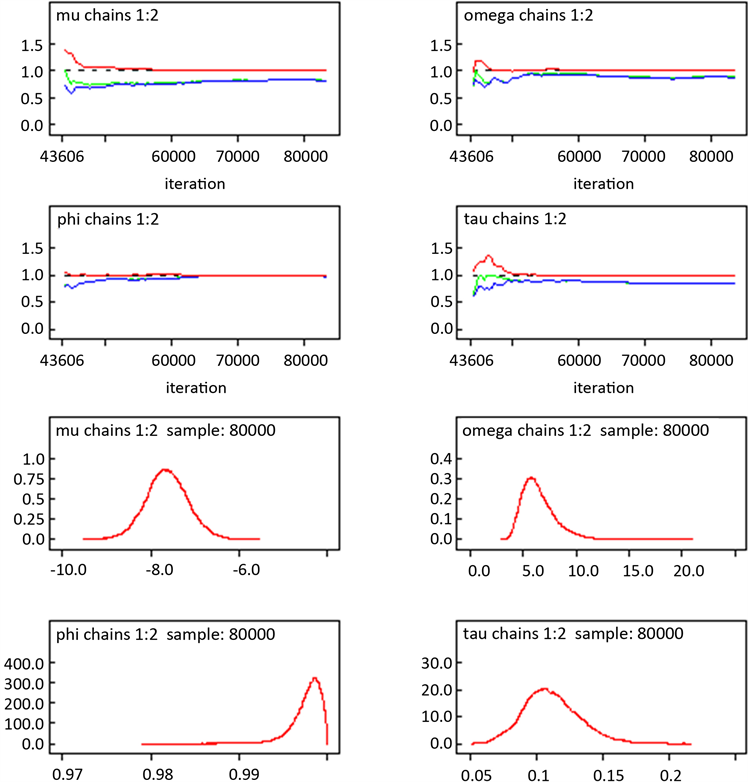Figure 7. bgr results of MCMC algorithm in SV-T model

5. 结论与建议

5.1. 主要结论

5.2. 主要建议

1) 信息披露等相关制度的健全要不断加强

2) 加强市场化建设，减少政府干预

3) 加强对投资者的教育

4) 加强证券市场法制建设

Volatility Analysis of Shenzhen and Shanghai Stock Markets—Based on GARCH and SV Models[J]. 社会科学前沿, 2019, 08(08): 1467-1476. https://doi.org/10.12677/ASS.2019.88200

1. 1. Engle, R.F. (1982) Autoregressive Conditional Heteroscedasticity with Estimates of the Variance of the United Kingdom Inflation. Econometrica, 50, 987-1007. https://doi.org/10.2307/1912773

2. 2. Bollersleve, T. (1986) Generalized Autoregressive Conditional Heteroskedasticity. Journal of Econometrics, 31, 307-327. https://doi.org/10.1016/0304-4076(86)90063-1

3. 3. Taylor, S.J. (1986) Modelling Financial Time Series. John Wiley & Sons, ‎Hoboken, NJ.

4. 4. 王悦. 基于GARCH模型的上证指数收益率波动性分析[J]. 纳税, 2018(5): 197.

5. 5. 宋华, 张锋. 深证综合指数收益率波动实证分析[J]. 吉林工商学院学报, 2018, 34(2): 70-74.

6. 6. 张鹤鸣. 上证指数收益率波动的实证分析[J]. 广西质量监督导报, 2019(2): 154-156.

7. 7. 王春峰, 姚守宇, 房振明. 收益率厚尾分布, 特质性波动与股票价格行为[J]. 系统工程, 2017, 35(12): 1-14.

8. 8. 谭璇. 基于GARCH模型族的中国股市波动率检测[J]. 武陵学刊, 2018, 43(6): 31-37.

9. 9. 王黎明. 应用时间序列分析[M]. 上海: 复旦大学出版社, 2009.

10. 10. 武倩雯. 上证指数收益率波动的实证分析: 基于ARCH族模型[J]. 区域金融研究, 2014(4): 42-48.